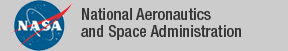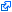### Top File of PVS numbers Library

```
top: THEORY
%-------------------------------------------------------------------------
% Numbers library
%
%     Authors:   Rick Butler       NASA Langley
%                Anthony Narkawicz NASA Langley
%                Alfons Geser      HTWK Leipzig, Germany
%-------------------------------------------------------------------------
BEGIN

IMPORTING
prime_factorization,   % factorization into primes
unique_factorization,  % factorization is unique
product_perm_lems,
infinite_primes,       % set of primes has infinite cardinality
sqrt_two,              % sqrt(2) irrational
eq_mod,
primes_sum_squares,    % every prime p with mod(p,4)=1 is a sum of two squares
chinese_remainder,
fermats_little_theorem % every prime p satisfies mod(a^p,p) = a for all a < p

END top
```
The tagidentifies links that are outside the NASA domain